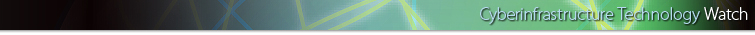November 2006 B
High Productivity Computing Systems and the Path Towards Usable Petascale Computing
Pedro C. Diniz, Information Sciences Institute, University of Southern California
Tejus Krishna, Information Sciences Institute, University of Southern California

5
4. Experimental Results

We now describe the utilization of the compiler analysis and code instrumentation infrastructure, described in the previous two sections, to several kernels codes, respectively, the `CGM` kernel from the NAS benchmark suite, a streaming kernel `streamadd`, and `randomaccess` from the HPCS benchmark suite. Whereas the spatial and temporal behavior of the HPCS kernels is fairly intuitive, the `CGM` kernel offers a mix of simple behaviors making it a richer example to experiment with.

4.1. The Kernels

The NAS CGM kernel is written in FORTRAN and implements the conjugate-gradient (CGM) iterative refinements method for a positive-definite input sparse-matrix. At the core of this kernel is the matvec subroutine that implements the sparse-matrix vector multiplication computation as depicted in the figure below.

``` 735:        subroutine matvec(n, a, rowidx, colstr, x, y) 736: c 737:        integer rowidx(1), colstr(1) 738:        integer n, i, j, k 739:        real*8 a(1), x(n), y(n), xj 740: c 741:        do 10 i = 1, n 742:          y(i) = 0.0d0 743:     10 continue 744:        do 200 j = 1, n 745:          xj = x(j) 746: 747:          do 100 k = colstr(j) , colstr(j+1)-1 748:            y(rowidx(k)) = y(rowidx(k)) + a(k) * xj 749:     100 continue 750:     200 continue 751:         return 752:         end ```

Figure 6. Source code for the CGM kernel.

The `streamadd` kernel is written in C and consists of a simple array vector addition loop. The length of the vector and thus of the manipulated arrays is fairly large, say 10,000 elements, to exercise the bandwidth of the memory subsystem. The `randomaccess` kernel, also known as the `gups` kernel, performs many updates to randomly selected locations in memory where the addresses of the locations are pre-computed in a loop before the updates take place. Figure 7 below depicts the relevant portions of the C source code for these kernels.

 ``` 08: for(i=0; i < N; i++){ 09: c[i] = a[i] + b[i]; 10: } ``` ``` 24: for(i=0; i < M; i++){ 25: loc[adder[i]]++; 26: } ```
Figure 7. Source code for the `streamadd` (left) and `randomaccess` (right) kernels.
4.2. Analysis Results

For each code, the analysis identifies the number of basic blocks and instruments the code to capture the corresponding frequency of execution. Table 1 below depicts the various results for the various kernels. Row two presents the number of basic blocks and row three the minimum, maximum and the average number of times one of these basic blocks executes. Row four presents the number and type of strides the static analysis determines whereas row five presents the corresponding spatial locality score. Row 6 shows the temporal locality score using the formulation presented in figure 4 above.

 `streamadd` `randomaccess` `NAS CGM` Number of Basic Blocks 5 12 14 Frequency of Execution [min, avg, max] [1:200:1,000] [1::2,000,000] [1:269,729:1,853,104] Stride and Reference Information 3 refs stride 1 1 ref stride 0; 2 refs random 2 refs random; 7 refs stride 1 Spatial Score 0.999 0.001 0.714 Temporal Score 0.1 0.000 0.422
Table 1. Experimental results for 3 code kernels.

Notice that the spatial score taking into account the frequency with which each basic block executes and thus although `CGM` has seven references with stride 1 in the various basic blocks of the core of this computation its spatial score is lower that the number of strided-1 references would otherwise suggest. This is due to the fact that some basic blocks with high spatial score execute very seldom.

Pages: 1 2 3 4 5 6